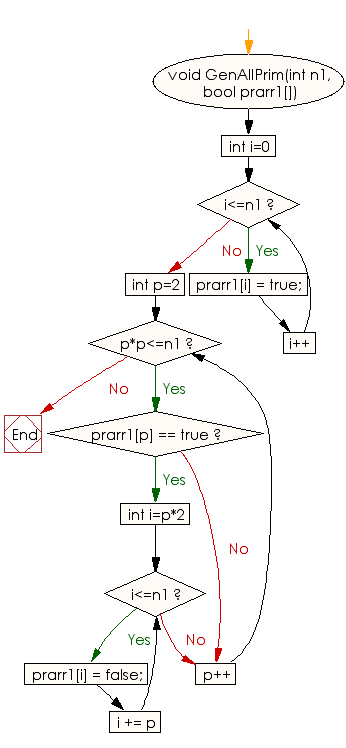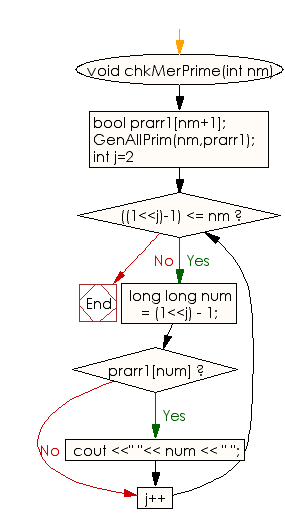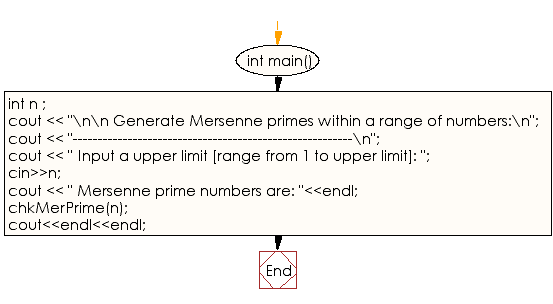﻿ C++ : Generate Mersenne primes within a range of numbers

# C++ Exercises: Generate Mersenne primes within a range of numbers

## C++ Numbers: Exercise-36 with Solution

Write a C++ program to generate Mersenne primes within a range of numbers.

Sample Solution:

C++ Code :

``````#include<bits/stdc++.h>
using namespace std;

void GenAllPrim(int n1, bool prarr1[])
{
for (int i=0; i<=n1; i++)
prarr1[i] = true;

for (int p=2; p*p<=n1; p++)
{
if (prarr1[p] == true)
{
for (int i=p*2; i<=n1; i += p)
prarr1[i] = false;
}
}
}
void chkMerPrime(int nm)
{
bool prarr1[nm+1];
GenAllPrim(nm,prarr1);
for (int j=2; ((1<<j)-1) <= nm; j++)
{
long long num = (1<<j) - 1;
if (prarr1[num])
cout <<" "<< num << " ";
}
}
int main()
{
int n ;
cout << "\n\n Generate Mersenne primes within a range of numbers:\n";
cout << "--------------------------------------------------------\n";
cout << " Input a upper limit [range from 1 to upper limit]: ";
cin>>n;
cout << " Mersenne prime numbers are: "<<endl;
chkMerPrime(n);
cout<<endl<<endl;
}
``````

Sample Output:

```Generate Mersenne primes within a range of numbers:
--------------------------------------------------------
Input a upper limit [range from 1 to upper limit]: 200
Mersenne prime numbers are:
3  7  31  127
```

Flowchart:C++ Code Editor:

What is the difficulty level of this exercise?

﻿

## C++ Programming: Tips of the Day

Why is there no std::stou?

The most pat answer would be that the C library has no corresponding "strtou", and the C++11 string functions are all just thinly veiled wrappers around the C library functions: The std::sto* functions mirror strto*, and the std::to_string functions use sprintf.

Ref: https://bit.ly/3wtz2qA

We are closing our Disqus commenting system for some maintenanace issues. You may write to us at reach[at]yahoo[dot]com or visit us at Facebook